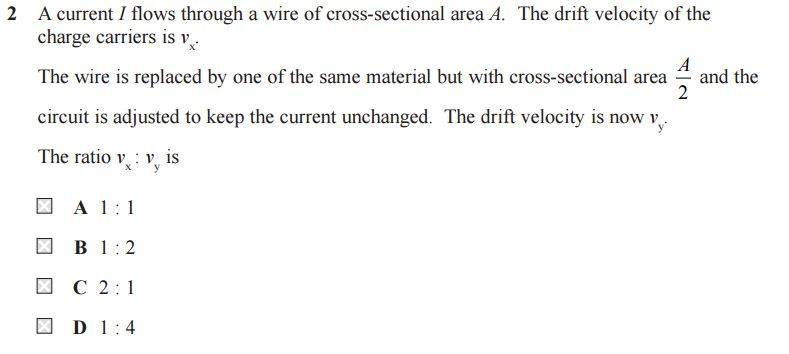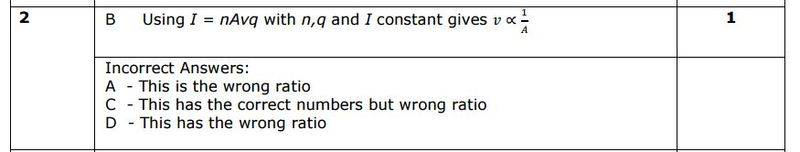# Charge carrier drift velocity of wire

## Homework Statement## The Attempt at a Solution

Current is I = nqvA so drift velocity V is: V = I/nqA

Drift velocity for x is: Vx = I/nqA

Drift velocity for y is: Vy = 2I/nqA

So the ratio of Vx : Vy should be 2:1 since Vy is equal to 2 lots of Vx?? (but correct answer is B)

gneill
Mentor
So the ratio of Vx : Vy should be 2:1 since Vy is equal to 2 lots of Vx?? (but correct answer is B)
You may want to rethink your logic there.

Edit: My mistake. I presumed that their table presented the ratios as ##V_y:V_x##, but looking again at the text I see that it's the other way around. So the book's suggested answer is incorrect as you found.

Edit2: I retract my retraction! See later postings.

Last edited:

## Homework Statement

View attachment 111644

## The Attempt at a Solution

Current is I = nqvA so drift velocity V is: V = I/nqA

Drift velocity for x is: Vx = I/nqA

Drift velocity for y is: Vy = 2I/nqA

So the ratio of Vx : Vy should be 2:1 since Vy is equal to 2 lots of Vx?? (but correct answer is B)

You may want to rethink your logic there.

Edit: My mistake. I presumed that their table presented the ratios as ##V_y:V_x##, but looking again at the text I see that it's the other way around. So the book's suggested answer is incorrect as you found.

I've done this question a few times now and ended up with the same answer, but the question is from an official exam paper in the UK so I don't believe there's been a mistake.

FYI, here's what the mark scheme says:How can it still be B?

gneill
Mentor
I'm retracting my edit from before. Clearly the coffee isn't working this morningIf the cross sectional area is halved the velocity doubles. So Vy is twice Vx as you originally pointed out:
since Vy is equal to 2 lots of Vx
So ##V_y## is twice the size of ##V_x##. That makes the ratio ##V_x:V_y = 1:2## which is indeed answer B.

I'm retracting my edit from before. Clearly the coffee isn't working this morningIf the cross sectional area is halved the velocity doubles. So Vy is twice Vx as you originally pointed out:

So ##V_y## is twice the size of ##V_x##. That the ratio ##V_x:V_y = 1:2## which is indeed answer B.

Indeed. Vy/2=Vx , so Vx: Vy should be 1:2 because then Vy÷2=1.

I'm retracting my edit from before. Clearly the coffee isn't working this morningIf the cross sectional area is halved the velocity doubles. So Vy is twice Vx as you originally pointed out:

So ##V_y## is twice the size of ##V_x##. That makes the ratio ##V_x:V_y = 1:2## which is indeed answer B.

Indeed. Vy/2=Vx , so Vx: Vy should be 1:2 because then Vy÷2=1.

Vx = I/nqA

and

Vy = 2[I/nqA] = 2Vx

so doesn't that mean that for every 1 part of Vx we have half a part of Vy? So that 2 parts of Vx gives 1 part of Vy so the ratio is still C, 2:1?? Argh!

gneill
Mentor
Pick a value for the magnitude of Vx, say 1. What value would you assign to Vy using your expressions?

Vx = I/nqA

and

Vy = 2[I/nqA] = 2Vx

so doesn't that mean that for every 1 part of Vx we have half a part of Vy? So that 2 parts of Vx gives 1 part of Vy so the ratio is still C, 2:1?? Argh!

2Vx=Vy. So , if the value of Vx is 1, value of Vy should be 2 right?

gneill
Mentor
2Vx=Vy. So , if the value of Vx is 1, value of Vy should be 2 right?
Right. So, Vx = 1, Vy = 2. What's Vx:Vy?

Right. So, Vx = 1, Vy = 2. What's Vx:Vy?

Then Vx:Vy should be 1:2